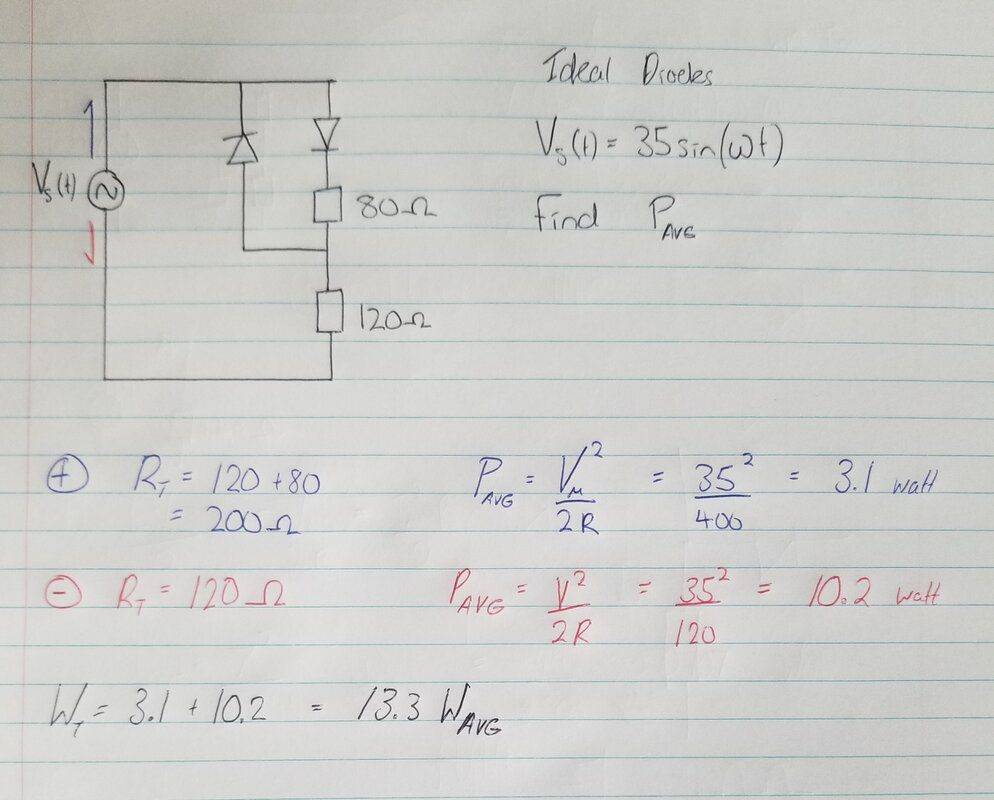# Beginner diode questions

WRS
New poster has been reminded to post schoolwork problems in the Homework Help forumsHello all,

I've just started learning about diodes.. I believe I've done this problem correctly (question in black), although want to make certain I'm understanding the very basics before moving on. Thank you

The applied voltage has a peak amplitude of ±35 volt.
You should be using the RMS voltage, for half the time.
So why did you use a factor of ½ in; P = V² / 2·R

Is this homework or private study?

Homework Helper
Gold Member
2022 Award
View attachment 279560

Hello all,

I've just started learning about diodes.. I believe I've done this problem correctly (question in black), although want to make certain I'm understanding the very basics before moving on. Thank you
You have found the average power during each half-cycle correctly.

However, adding the two powers is wrong. I’ll make up a simple example for illustration:
Frequency = 1Hz (So each half-cycle takes 0.5s)
Average power during 1st half of cycle = 8W
Average power during 2nd half of cycle = 20W
Energy/cycle = 8*0.5 + 20*0.5 = 14J
Average power during complete cycle = ##\frac {14J}{1s} = 14W## (not 28W).

Note to @Baluncore
$$P_{avg} = \frac {V_{peak}^2}{2R}$$
is fine because the average power is half the peak power.
This will be clear if you apply ##V_{peak} = {V_{rms} √2}##

•DaveE
You have found the average power during each half-cycle correctly.
@Steve4Physics
is fine because the average power is half the peak power.
You cannot be sure if the factor of ½ employed by the student was for the RMS conversion, or for the 50% duty cycle conversion. For that reason we should not do the students homework, but should ask the student to explain their derivation of their equation. @WRS got it half correct.

•Steve4Physics
Homework Helper
Gold Member
2022 Award
@Steve4Physics

You cannot be sure if the factor of ½ employed by the student was for the RMS conversion, or for the 50% duty cycle conversion. For that reason we should not do the students homework, but should ask the student to explain their derivation of their equation. @WRS got it half correct.
I see what you mean. Since the OP had written$$P_{AVG} = \frac {V_M^2}{2R}$$I assumed ##V_M## was intended to be the maximum (peak) voltage, so thie formula simply appeared to be a statement of a ‘standard’ result.

However, I agree that it is quite possible that the OP had misunderstood. It would have been better to let him clarify this.

•Baluncore